# Fault analysis of simple circuits

## Electricity and Electronics

• #### Question 1

Suppose a voltmeter registers 0 volts between test points {\bf C} and {\bf F}, but measures 24 volts between those same test points when the connection between {\bf B} and {\bf C} is broken: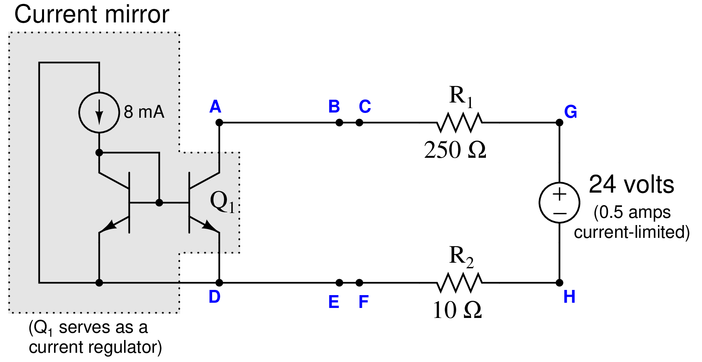Identify the likelihood of each specified fault for this circuit. Consider each fault one at a time (i.e. no coincidental faults), determining whether or not each fault could independently account for {\it all} measurements and symptoms in this circuit.

$$\begin{array} {|l|l|} \hline Fault & Possible & Impossible \\ \hline R_1 failed open & & \\ \hline R_2 failed open & & \\ \hline Q_1 failed open & & \\ \hline R_1 failed shorted & & \\ \hline R_2 failed shorted & & \\ \hline Q_1 failed shorted & & \\ \hline Voltage source dead & & \\ \hline \end{array}$$

• #### Question 2

Suppose this electric-driven air compressor cycles between 60 PSI and 105 PSI when the switch is in the Auto’’ position, and cycles between 102 PSI and 105 PSI when in the Hand’’ position: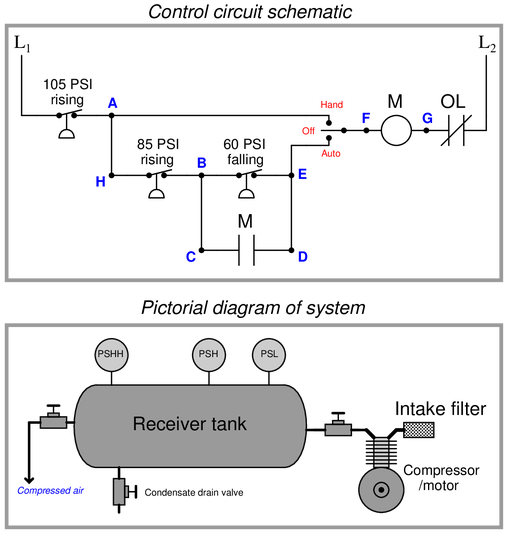Identify the likelihood of each specified fault for this circuit. Consider each fault one at a time (i.e. no coincidental faults), determining whether or not each fault could independently account for {\it all} measurements and symptoms in this circuit.

$$\begin{array} {|l|l|} \hline Fault & Possible & Impossible \\ \hline PSHH failed open & & \\ \hline PSH failed open & & \\ \hline PSL failed open & & \\ \hline Auxiliary M’’ contact failed open & & \\ \hline PSHH failed shorted & & \\ \hline PSH failed shorted & & \\ \hline PSL failed shorted & & \\ \hline Auxiliary M’’ contact failed shorted & & \\ \hline \end{array}$$

• #### Question 3

Suppose this electric-driven air compressor refuses to start when the switch is in the Auto’’ position, but starts up immediately when the switch is placed in the Hand’’ position. The first test performed by a technician is to measure AC voltage between test points {\bf A} and {\bf F} with the switch in the Auto’’ position. There, the meter registers 117 volts AC. You are then called in to help:Identify the likelihood of each specified fault for this circuit. Consider each fault one at a time (i.e. no coincidental faults), determining whether or not each fault could independently account for {\it all} measurements and symptoms in this circuit.

$$\begin{array} {|l|l|} \hline Fault & Possible & Impossible \\ \hline PSHH failed open & & \\ \hline PSH failed open & & \\ \hline PSL failed open & & \\ \hline Hand’’ switch position failed open & & \\ \hline Auto’’ switch position failed open & & \\ \hline OL contact failed open & & \\ \hline Auxiliary M’’ contact failed & & \\ \hline Contactor M’’ coil failed open & & \\ \hline \end{array}$$

• #### Question 4

An Automation Direct model C0-08TD2 sourcing’’ DC output PLC module uses the following internal circuitry to switch DC power to a load: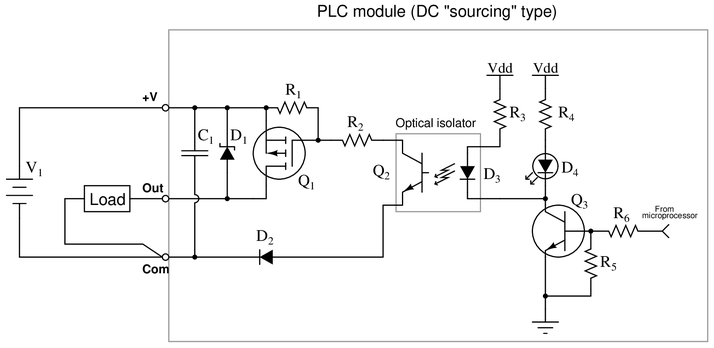Suppose the microprocessor is sending a high’’ (1) signal to the switching circuitry, but the DC load refuses to energize. Using your DC voltmeter, you measure 24.7 volts DC between the +V’’ and Com’’ terminals, and 0 volts DC between the Out’’ and Com’’ terminals.

Identify the likelihood of each specified fault for this circuit. Consider each fault one at a time (i.e. no coincidental faults), determining whether or not each fault could independently account for {\it all} measurements and symptoms in this circuit.

$$\begin{array} {|l|l|} \hline Fault & Possible & Impossible \\ \hline Diode D_1 failed shorted & & \\ \hline Diode D_2 failed open & & \\ \hline Diode D_3 failed open & & \\ \hline Diode D_4 failed open & & \\ \hline Transistor Q_1 failed open & & \\ \hline Transistor Q_2 failed open & & \\ \hline Transistor Q_3 failed shorted & & \\ \hline Capacitor C_1 failed open & & \\ \hline Resistor R_1 failed shorted & & \\ \hline Resistor R_2 failed shorted & & \\ \hline Resistor R_3 failed open & & \\ \hline Resistor R_4 failed open & & \\ \hline Resistor R_5 failed shorted & & \\ \hline Resistor R_6 failed open & & \\ \hline \end{array}$$

• #### Question 5

An Automation Direct model C0-08TD1 sinking’’ DC output PLC module uses the following internal circuitry to switch DC power to a load: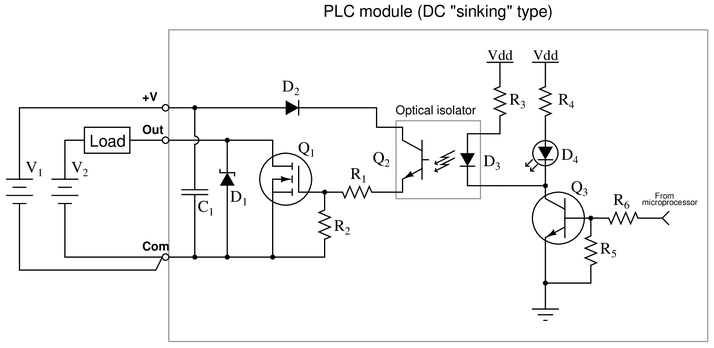Suppose the microprocessor is sending a high’’ (1) signal to the switching circuitry, but the DC load refuses to energize. Using your DC voltmeter, you measure 24.7 volts DC between the +V’’ and Com’’ terminals, and 23.2 volts DC between the Out’’ and Com’’ terminals.

Identify the likelihood of each specified fault for this circuit. Consider each fault one at a time (i.e. no coincidental faults), determining whether or not each fault could independently account for {\it all} measurements and symptoms in this circuit.

$$\begin{array} {|l|l|} \hline Fault & Possible & Impossible \\ \hline Diode D_1 failed open & & \\ \hline Diode D_2 failed open & & \\ \hline Diode D_3 failed open & & \\ \hline Diode D_4 failed open & & \\ \hline Transistor Q_1 failed open & & \\ \hline Transistor Q_2 failed open & & \\ \hline Transistor Q_3 failed open & & \\ \hline Resistor R_1 failed open & & \\ \hline Resistor R_2 failed open & & \\ \hline Resistor R_3 failed open & & \\ \hline Resistor R_4 failed open & & \\ \hline Resistor R_5 failed open & & \\ \hline Resistor R_6 failed open & & \\ \hline \end{array}$$

• #### Question 6

Suppose the voltmeter in this circuit registers a strong {\it negative} voltage. A test using a digital multimeter (DMM) shows the voltage between test points {\bf A} and {\bf B} to be 0.8 volts: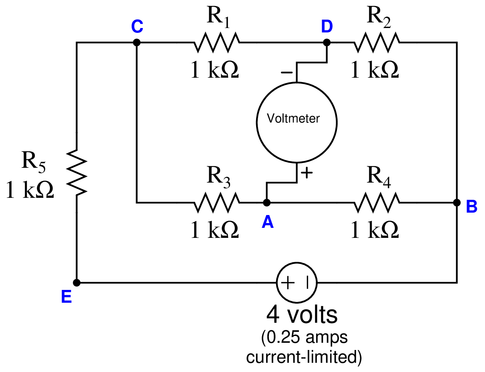Identify the likelihood of each specified fault for this circuit. Consider each fault one at a time (i.e. no coincidental faults), determining whether or not each fault could independently account for {\it all} measurements and symptoms in this circuit.

$$\begin{array} {|l|l|} \hline Fault & Possible & Impossible \\ \hline R_1 failed open & & \\ \hline R_2 failed open & & \\ \hline R_3 failed open & & \\ \hline R_4 failed open & & \\ \hline R_5 failed open & & \\ \hline R_1 failed shorted & & \\ \hline R_2 failed shorted & & \\ \hline R_3 failed shorted & & \\ \hline R_4 failed shorted & & \\ \hline R_5 failed shorted & & \\ \hline Voltage source dead & & \\ \hline \end{array}$$

• #### Question 7

Suppose the voltmeter in this bridge circuit registers a strong {\it negative} voltage. A test using a digital multimeter (DMM) shows the voltage between test points {\bf A} and {\bf C} to be 4 volts: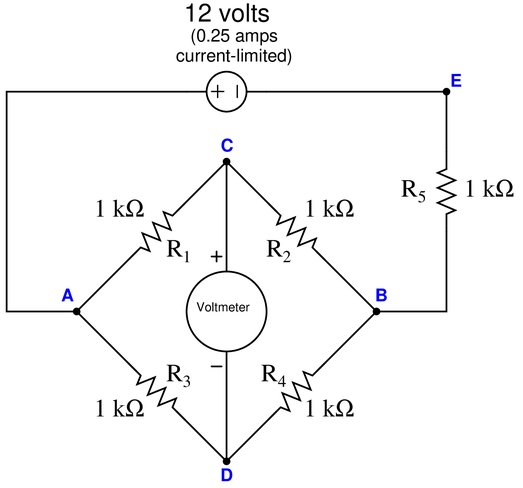Identify the likelihood of each specified fault for this circuit. Consider each fault one at a time (i.e. no coincidental faults), determining whether or not each fault could independently account for {\it all} measurements and symptoms in this circuit.

$$\begin{array} {|l|l|} \hline Fault & Possible & Impossible \\ \hline R_1 failed open & & \\ \hline R_2 failed open & & \\ \hline R_3 failed open & & \\ \hline R_4 failed open & & \\ \hline R_5 failed open & & \\ \hline R_1 failed shorted & & \\ \hline R_2 failed shorted & & \\ \hline R_3 failed shorted & & \\ \hline R_4 failed shorted & & \\ \hline R_5 failed shorted & & \\ \hline Voltage source dead & & \\ \hline \end{array}$$

• #### Question 8

Suppose the voltmeter in this bridge circuit registers a strong {\it positive} voltage. A test using a digital multimeter (DMM) shows the voltage between test points {\bf A} and {\bf B} to be 12 volts:Identify the likelihood of each specified fault for this circuit. Consider each fault one at a time (i.e. no coincidental faults), determining whether or not each fault could independently account for {\it all} measurements and symptoms in this circuit.

$$\begin{array} {|l|l|} \hline Fault & Possible & Impossible \\ \hline R_1 failed open & & \\ \hline R_2 failed open & & \\ \hline R_3 failed open & & \\ \hline R_4 failed open & & \\ \hline R_1 failed shorted & & \\ \hline R_2 failed shorted & & \\ \hline R_3 failed shorted & & \\ \hline R_4 failed shorted & & \\ \hline Voltage source dead & & \\ \hline \end{array}$$

• #### Question 9

Suppose the voltmeter in this bridge circuit registers a strong {\it negative} voltage: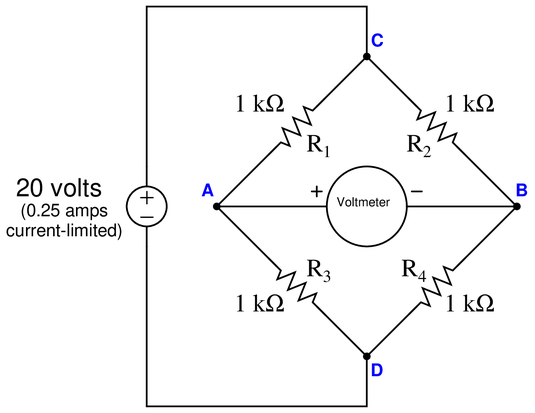Identify the likelihood of each specified fault for this circuit. Consider each fault one at a time (i.e. no coincidental faults), determining whether or not each fault could independently account for {\it all} measurements and symptoms in this circuit.

$$\begin{array} {|l|l|} \hline Fault & Possible & Impossible \\ \hline R_1 failed open & & \\ \hline R_2 failed open & & \\ \hline R_3 failed open & & \\ \hline R_4 failed open & & \\ \hline R_1 failed shorted & & \\ \hline R_2 failed shorted & & \\ \hline R_3 failed shorted & & \\ \hline R_4 failed shorted & & \\ \hline Voltage source dead & & \\ \hline \end{array}$$

• #### Question 10

Suppose a voltmeter registers 22 volts between test points {\bf A} and {\bf D}: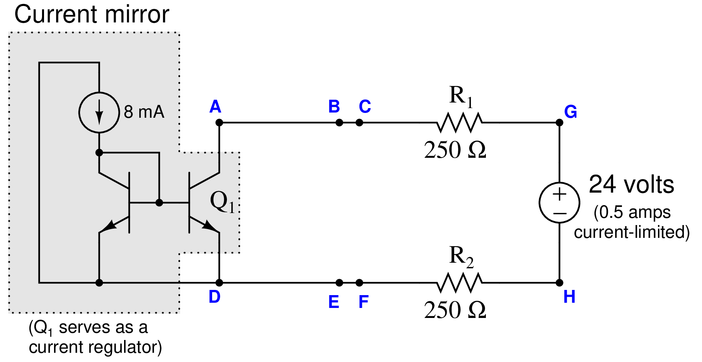Identify the likelihood of each specified fault for this circuit. Consider each fault one at a time (i.e. no coincidental faults), determining whether or not each fault could independently account for {\it all} measurements and symptoms in this circuit.

$$\begin{array} {|l|l|} \hline Fault & Possible & Impossible \\ \hline R_1 failed open & & \\ \hline R_2 failed open & & \\ \hline Q_1 failed open & & \\ \hline R_1 failed shorted & & \\ \hline R_2 failed shorted & & \\ \hline Q_1 failed shorted & & \\ \hline Voltage source dead & & \\ \hline \end{array}$$

• #### Question 11

This pictorial diagram shows the wiring connections for a simple pressure control loop, where a loop-powered 4-20 mA pressure transmitter sends a signal to a Honeywell controller, which in turn sends another 4-20 mA signal to a control valve: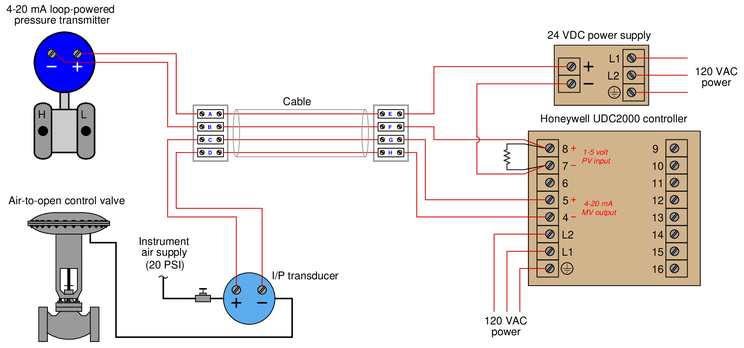If an operator informs you that the pressure indicated by the Honeywell controller is below range (pegged’’ full downscale, reading -10

• #### Question 12

Suppose a voltmeter registers 6 volts between test points {\bf C} and {\bf D} while the pushbutton is released (not pressed), and also 6 volts between the same test points while the pushbutton is pressed:Identify the likelihood of each specified fault for this circuit. Consider each fault one at a time (i.e. no coincidental faults), determining whether or not each fault could independently account for {\it all} measurements and symptoms in this circuit.

$$\begin{array} {|l|l|} \hline Fault & Possible & Impossible \\ \hline Switch failed open & & \\ \hline R_1 failed open & & \\ \hline R_2 failed open & & \\ \hline R_3 failed open & & \\ \hline Switch failed shorted & & \\ \hline R_1 failed shorted & & \\ \hline R_2 failed shorted & & \\ \hline R_3 failed shorted & & \\ \hline Voltage source dead & & \\ \hline \end{array}$$

• #### Question 13

Suppose a voltmeter registers 24 volts between test points {\bf E} and {\bf C} while the pushbutton is released (not pressed), and 0 volts between test points {\bf A} and {\bf B} while the pushbutton is pressed:Identify the likelihood of each specified fault for this circuit. Consider each fault one at a time (i.e. no coincidental faults), determining whether or not each fault could independently account for {\it all} measurements and symptoms in this circuit.

$$\begin{array} {|l|l|} \hline Fault & Possible & Impossible \\ \hline Switch failed open & & \\ \hline R_1 failed open & & \\ \hline R_2 failed open & & \\ \hline R_3 failed open & & \\ \hline Switch failed shorted & & \\ \hline R_1 failed shorted & & \\ \hline R_2 failed shorted & & \\ \hline R_3 failed shorted & & \\ \hline Voltage source dead & & \\ \hline \end{array}$$

• #### Question 14

Suppose a voltmeter registers 0 volts between test points {\bf E} and {\bf F}, and 9 volts between test points {\bf F} and {\bf H} when the connection between {\bf F} and {\bf H} has been broken:Identify the likelihood of each specified fault for this circuit. Consider each fault one at a time (i.e. no coincidental faults), determining whether or not each fault could independently account for {\it all} measurements and symptoms in this circuit.

$$\begin{array} {|l|l|} \hline Fault & Possible & Impossible \\ \hline R_1 failed open & & \\ \hline R_2 failed open & & \\ \hline R_3 failed open & & \\ \hline R_1 failed shorted & & \\ \hline R_2 failed shorted & & \\ \hline R_3 failed shorted & & \\ \hline Voltage source dead & & \\ \hline \end{array}$$

• #### Question 15

Suppose a voltmeter registers 0 volts between test points {\bf B} and {\bf C}, and measures 24 volts between those same two test points after the connection has been broken between points {\bf A} and {\bf B}:Identify the likelihood of each specified fault for this circuit. Consider each fault one at a time (i.e. no coincidental faults), determining whether or not each fault could independently account for {\it all} measurements and symptoms in this circuit.

$$\begin{array} {|l|l|} \hline Fault & Possible & Impossible \\ \hline R_1 failed open & & \\ \hline R_2 failed open & & \\ \hline R_3 failed open & & \\ \hline R_4 failed open & & \\ \hline R_1 failed shorted & & \\ \hline R_2 failed shorted & & \\ \hline R_3 failed shorted & & \\ \hline R_4 failed shorted & & \\ \hline Voltage source dead & & \\ \hline \end{array}$$

• #### Question 16

Suppose a voltmeter registers 9 volts between test points {\bf B} and {\bf C}, but measures 18 volts between those same two test points after the connection has been broken between points {\bf E} and {\bf G}:Identify the likelihood of each specified fault for this circuit. Consider each fault one at a time (i.e. no coincidental faults), determining whether or not each fault could independently account for {\it all} measurements and symptoms in this circuit.

$$\begin{array} {|l|l|} \hline Fault & Possible & Impossible \\ \hline R_1 failed open & & \\ \hline R_2 failed open & & \\ \hline R_3 failed open & & \\ \hline R_1 failed shorted & & \\ \hline R_2 failed shorted & & \\ \hline R_3 failed shorted & & \\ \hline Voltage source dead & & \\ \hline \end{array}$$

• #### Question 17

Suppose a voltmeter registers 0 volts between test points {\bf A} and {\bf C}, and also measures 0 volts between those same two test points after the connection has been broken between points {\bf A} and {\bf B}:Identify the likelihood of each specified fault for this circuit. Consider each fault one at a time (i.e. no coincidental faults), determining whether or not each fault could independently account for {\it all} measurements and symptoms in this circuit.

$$\begin{array} {|l|l|} \hline Fault & Possible & Impossible \\ \hline R_1 failed open & & \\ \hline R_2 failed open & & \\ \hline R_3 failed open & & \\ \hline R_1 failed shorted & & \\ \hline R_2 failed shorted & & \\ \hline R_3 failed shorted & & \\ \hline Voltage source dead & & \\ \hline \end{array}$$

• #### Question 18

Suppose an ammeter inserted between test point {\bf C} and the nearest lead of resistor R_2 registers 0 mA in this series-parallel circuit:Identify the likelihood of each specified fault for this circuit. Consider each fault one at a time (i.e. no coincidental faults), determining whether or not each fault could independently account for {\it all} measurements and symptoms in this circuit.

$$\begin{array} {|l|l|} \hline Fault & Possible & Impossible \\ \hline R_1 failed open & & \\ \hline R_2 failed open & & \\ \hline R_3 failed open & & \\ \hline R_4 failed open & & \\ \hline R_1 failed shorted & & \\ \hline R_2 failed shorted & & \\ \hline R_3 failed shorted & & \\ \hline R_4 failed shorted & & \\ \hline Current source dead & & \\ \hline \end{array}$$

• #### Question 19

Suppose an ammeter inserted between test point {\bf F} and the nearest lead of resistor R_4 registers 0 mA in this series-parallel circuit:Identify the likelihood of each specified fault for this circuit. Consider each fault one at a time (i.e. no coincidental faults), determining whether or not each fault could independently account for {\it all} measurements and symptoms in this circuit.

$$\begin{array} {|l|l|} \hline Fault & Possible & Impossible \\ \hline R_1 failed open & & \\ \hline R_2 failed open & & \\ \hline R_3 failed open & & \\ \hline R_4 failed open & & \\ \hline R_1 failed shorted & & \\ \hline R_2 failed shorted & & \\ \hline R_3 failed shorted & & \\ \hline R_4 failed shorted & & \\ \hline Current source dead & & \\ \hline \end{array}$$

• #### Question 20

Suppose a voltmeter registers 0 volts between test points {\bf A} and {\bf D} in this series-parallel circuit:Determine the diagnostic value of each of the following tests. Assume only one fault in the system, including any single component or any single wire/cable/tube connecting components together. If a proposed test could provide new information to help you identify the location and/or nature of the one fault, mark yes.’’ Otherwise, if a proposed test would not reveal anything relevant to identifying the fault (already discernible from the measurements and symptoms given so far), mark no.’‘

$$\begin{array} {|l|l|} \hline Diagnostic test & Yes & No \\ \hline Measure V_{CD} with power applied & & \\ \hline Measure V_{DF} with power applied & & \\ \hline Measure V_{DE} with power applied & & \\ \hline Measure I_{R1} with power applied & & \\ \hline Measure I_{R2} with power applied & & \\ \hline Measure R_{AB} with source disconnected from C & & \\ \hline Measure R_{DF} with source disconnected from E & & \\ \hline Measure R_{CF} with source disconnected from C & & \\ \hline Measure R_{CD} with wire disconnected between {\bf A} and C & & \\ \hline Measure R_{CD} with R_3 disconnected from D & & \\ \hline \end{array}$$

• #### Question 21

Suppose an operator discovers that the natural gas make-up valve in this fuel gas pressure control system is shut when the controller output is 78{\bullet} {\bf Test 1:} Measured 18.6 volts DC between PT-528 transmitter terminals.

{\bullet} {\bf Test 2:} Measured supply air pressure at PV-528a to be 22 PSIG.

{\bullet} {\bf Test 3:} Measured 0 volts DC between terminals 25 and 26.

{\bullet} {\bf Test 4:} Measured 27 volts DC between terminals 5 and 6.

{\bullet} {\bf Test 5:} Measured 27 volts DC between terminals 19 and 20.

{\bullet} {\bf Test 6:} Measured 27 volts DC between terminals 15 and 16.

Identify any useful information about the nature or location of the fault derived from the results of each test, in order of the tests performed. If the test is not useful (i.e. provides no new information), mark it as such. Assuming there is only one fault in the circuit, identify the location and nature of the fault as precisely as you can from the test results shown above.

• #### Question 22

This reversing motor control circuit has a problem: it runs just fine in reverse, but not at all in the forward direction. A technician begins diagnosing the circuit, following the steps shown (in order):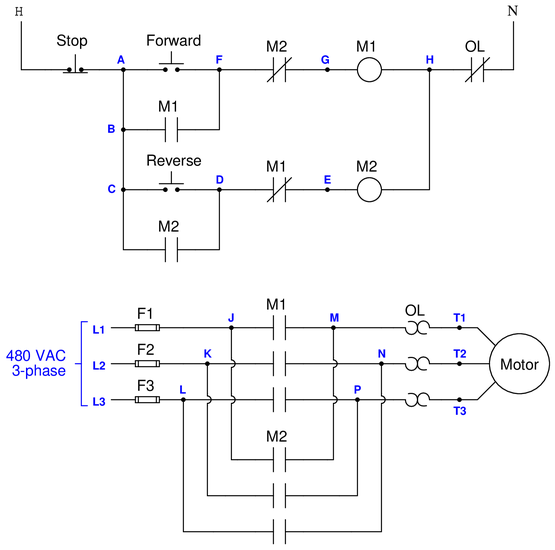{\bullet} {\bf Test 1:} Jumpered points {\bf F} and {\bf G} while pressing the Forward’’ pushbutton. Motor did not turn.

{\bullet} {\bf Test 2:} Measured 117 VAC between points {\bf G} and {\bf H} while pressing Forward’’ pushbutton.

{\bullet} {\bf Test 3:} Measured 478 VAC between points {\bf M} and {\bf N} while pressing Forward’’ pushbutton.

{\bullet} {\bf Test 4:} Measured 239 VAC between points {\bf M} and {\bf P} while pressing Forward’’ pushbutton.

{\bullet} {\bf Test 5:} Measured 239 VAC between points {\bf N} and {\bf P} while pressing Forward’’ pushbutton.

Identify any useful information about the nature or location of the fault derived from the results of each test, in order of the tests performed. If the test is not useful (i.e. provides no new information), mark it as such. Assuming there is only one fault in the circuit, identify the location and nature of the fault as precisely as you can from the test results shown above.

• #### Question 23

This conveyor belt control circuit has a problem. The siren energizes when the Start’’ pushbutton is pressed, but the conveyor belt never moves. The siren remains energized until the Stop’’ button is pressed. A technician begins diagnosing the circuit, following the steps shown (in order). His first action is to press the Start’’ switch so that the siren is continuously activated, before he begins any diagnostic tests:{\bullet} {\bf Test 1:} Measured 120 VAC between points {\bf A} and {\bf E}.

{\bullet} {\bf Test 2:} Measured 120 VAC between points {\bf N} and {\bf L}.

{\bullet} {\bf Test 3:} Measured 0 VAC between points {\bf J} and {\bf K}.

{\bullet} {\bf Test 4:} Measured 0 VAC between points {\bf D} and {\bf M}.

{\bullet} {\bf Test 5:} Measured 120 VAC between points {\bf A} and {\bf D}.

Identify any useful information about the nature or location of the fault derived from the results of each test, in order of the tests performed. If the test is not useful (i.e. provides no new information), mark it as such. Assuming there is only one fault in the circuit, identify the location and nature of the fault as precisely as you can from the test results shown above.

• #### Question 24

This air compressor control circuit has a problem. The air compressor refuses to start even when the air pressure is zero PSI. A technician begins diagnosing the circuit, following the steps shown (in order):{\bullet} {\bf Test 1:} Measured 120 VAC between points {\bf A} and {\bf C}, with Hand/Off/Auto’’ switch in the Auto’’ position.

{\bullet} {\bf Test 2:} Measured 120 VAC between points {\bf A} and {\bf D}, with Hand/Off/Auto’’ switch in Auto’’ position.

{\bullet} {\bf Test 3:} Measured 0 VAC between points {\bf E} and {\bf C}, with Hand/Off/Auto’’ switch in Auto’’ position.

{\bullet} {\bf Test 4:} Jumpered points {\bf A} and {\bf B}, with Hand/Off/Auto’’ switch in Auto’’ position. The motor did not start.

Identify any useful information about the nature or location of the fault derived from the results of each test, in order of the tests performed. If the test is not useful (i.e. provides no new information), mark it as such. Assuming there is only one fault in the circuit, identify the location and nature of the fault as precisely as you can from the test results shown above.

• #### Question 25

Suppose the electric motor refuses to run when the Run’’ pushbutton switch is pressed, whether the speed switch is set to Fast’’ or to Slow.’’ A technician begins diagnosing the circuit, following the steps shown (in order):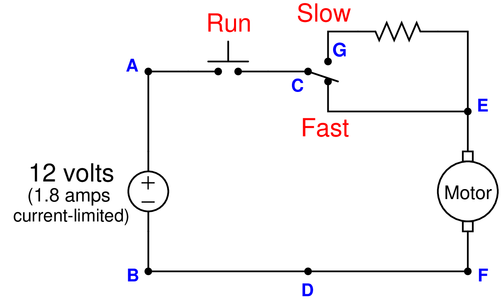{\bullet} {\bf Test 1:} Measured 12 volts DC between points {\bf A} and {\bf B}, with Run’’ switch pressed and speed switch in Fast’’ position.

{\bullet} {\bf Test 2:} Measured 0 volts DC between points {\bf A} and {\bf C}, with Run’’ switch unpressed and speed switch in Fast’’ position.

{\bullet} {\bf Test 3:} Measured 12 volts DC between points {\bf G} and {\bf D}, with Run’’ switch pressed and speed switch in Fast’’ position.

{\bullet} {\bf Test 4:} Measured 25 ohms between points {\bf G} and {\bf E}, with Run’’ switch unpressed and speed switch in Fast’’ position.

{\bullet} {\bf Test 5:} Measured 12 volts DC between points {\bf A} and {\bf F}, with Run’’ switch pressed and speed switch in Fast’’ position.

Identify any useful information about the nature or location of the fault derived from the results of each test, in order of the tests performed. If the test is not useful (i.e. provides no new information), mark it as such. Assuming there is only one fault in the circuit, identify the location and nature of the fault as precisely as you can from the test results shown above.

• #### Question 26

Suppose the electric motor refuses to run when the Run’’ pushbutton switch is pressed. A technician begins diagnosing the circuit, following the steps shown (in order):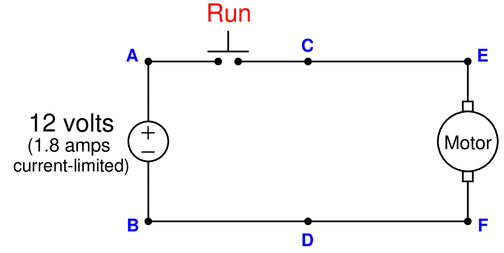{\bullet} {\bf Test 1:} Measured 0 volts DC between points {\bf C} and {\bf D}, with Run’’ switch pressed.

{\bullet} {\bf Test 2:} Measured 0 volts DC between points {\bf A} and {\bf C}, with Run’’ switch unpressed.

{\bullet} {\bf Test 3:} Measured 12 volts DC between points {\bf A} and {\bf B}, with Run’’ switch pressed.

{\bullet} {\bf Test 4:} Measured 12 volts DC between points {\bf C} and {\bf B}, with Run’’ switch unpressed.

{\bullet} {\bf Test 5:} Measured 3.5 ohms between points {\bf E} and {\bf F}, with Run’’ switch unpressed.

Identify any useful information about the nature or location of the fault derived from the results of each test, in order of the tests performed. If the test is not useful (i.e. provides no new information), mark it as such. Assuming there is only one fault in the circuit, identify the location and nature of the fault as precisely as you can from the test results shown above.

• #### Question 27

Suppose the electric motor refuses to run when the Run’’ pushbutton switch is pressed. A technician begins diagnosing the circuit, following the steps shown (in order):{\bullet} {\bf Test 1:} Measured 2.8 ohms between points {\bf E} and {\bf F}, with Run’’ switch unpressed.

{\bullet} {\bf Test 2:} Measured 12 volts between points {\bf A} and {\bf F}, with Run’’ switch unpressed.

{\bullet} {\bf Test 3:} Measured 12 volts between points {\bf A} and {\bf B}, with Run’’ switch unpressed.

{\bullet} {\bf Test 4:} Measured 12 volts between points {\bf A} and {\bf C}, with Run’’ switch pressed.

Identify any useful information about the nature or location of the fault derived from the results of each test, in order of the tests performed. If the test is not useful (i.e. provides no new information), mark it as such. Assuming there is only one fault in the circuit, identify the location and nature of the fault as precisely as you can from the test results shown above.

• #### Question 28

This pictorial diagram shows the wiring connections for a simple pressure control loop, where a loop-powered 4-20 mA pressure transmitter sends a signal to a Honeywell controller, which in turn sends another 4-20 mA signal to a control valve: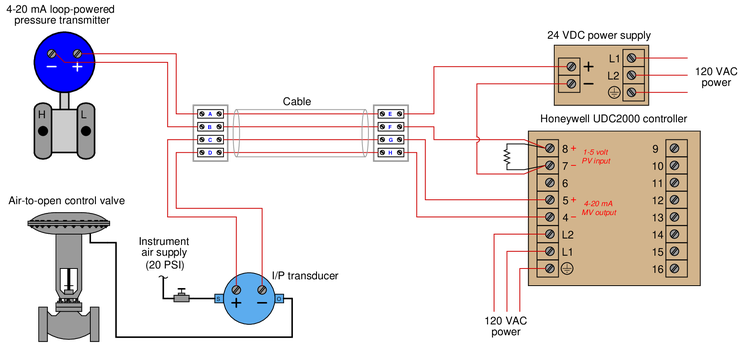Suppose the operator informs you that the control valve refuses to open, no matter what value she sets the output of the controller in manual mode. Your job now is to diagnose the problem in this control loop using only basic test equipment (e.g. digital multimeter, hand tools).

Determine the diagnostic value of each of the following tests. Assume only one fault in the system, including any single component or any single wire/cable/tube connecting components together. If a proposed test could provide new information to help you identify the location and/or nature of the one fault, mark yes.’’ Otherwise, if a proposed test would not reveal anything relevant to identifying the fault (already discernible from the measurements and symptoms given so far), mark no.’‘

$$\begin{array} {|l|l|} \hline Diagnostic test & Yes & No \\ \hline Place controller in automatic mode & & \\ \hline Measure V_{AB} with controller output set to 100\Measure V_{5-4} with controller output set to 100\Measure V_{8-7} with controller output set to 50\Crack’’ open tube fitting at the S’’ port on the I/P transducer & & \\ \hline Crack’’ open tube fitting at the O’’ port on the I/P transducer & & \\ \hline Press the I/P transducer’s flapper closer to its nozzle & & \\ \hline Pull the I/P transducer’s flapper away from its nozzle & & \\ \hline Tighten the nuts compressing the control valve’s stem packing & & \\ \hline Loosen the nuts compressing the control valve’s stem packing & & \\ \hline Measure the output voltage of the DC power supply & & \\ \hline Measure voltage across the pressure transmitter terminals & & \\ \hline Measure voltage across the I/P transducer terminals & & \\ \hline \end{array}$$

• #### Question 29

Suppose a voltmeter registers 0 volts between test points {\bf E} and {\bf F} in this circuit:Determine the diagnostic value of each of the following tests. Assume only one fault in the system, including any single component or any single wire/cable/tube connecting components together. If a proposed test could provide new information to help you identify the location and/or nature of the one fault, mark yes.’’ Otherwise, if a proposed test would not reveal anything relevant to identifying the fault (already discernible from the measurements and symptoms given so far), mark no.’‘

$$\begin{array} {|l|l|} \hline Diagnostic test & Yes & No \\ \hline Measure V_{AC} with power applied & & \\ \hline Measure V_{JK} with power applied & & \\ \hline Measure V_{CK} with power applied & & \\ \hline Measure I_{R1} with power applied & & \\ \hline Measure I_{R2} with power applied & & \\ \hline Measure I_{R3} with power applied & & \\ \hline Measure R_{AC} with source disconnected from R_1 & & \\ \hline Measure R_{DF} with source disconnected from R_1 & & \\ \hline Measure R_{EG} with source disconnected from R_1 & & \\ \hline Measure R_{HK} with source disconnected from R_1 & & \\ \hline \end{array}$$

• #### Question 30

Suppose a voltmeter registers 24 volts between test points {\bf A} and {\bf B} in this series-parallel circuit:Identify the likelihood of each specified fault for this circuit. Consider each fault one at a time (i.e. no coincidental faults), determining whether or not each fault could independently account for {\it all} measurements and symptoms in this circuit.

$$\begin{array} {|l|l|} \hline Fault & Possible & Impossible \\ \hline R_1 failed open & & \\ \hline R_2 failed open & & \\ \hline R_3 failed open & & \\ \hline R_4 failed open & & \\ \hline R_1 failed shorted & & \\ \hline R_2 failed shorted & & \\ \hline R_3 failed shorted & & \\ \hline R_4 failed shorted & & \\ \hline Current source dead & & \\ \hline \end{array}$$

• #### Question 31

Suppose a voltmeter registers 16 volts between test points {\bf C} and {\bf E} in this series-parallel circuit:Determine the diagnostic value of each of the following tests. Assume only one fault in the system, including any single component or any single wire/cable/tube connecting components together. If a proposed test could provide new information to help you identify the location and/or nature of the one fault, mark yes.’’ Otherwise, if a proposed test would not reveal anything relevant to identifying the fault (already discernible from the measurements and symptoms given so far), mark no.’‘

$$\begin{array} {|l|l|} \hline Diagnostic test & Yes & No \\ \hline Measure V_{CD} with power applied & & \\ \hline Measure V_{DF} with power applied & & \\ \hline Measure I_{R1} with power applied & & \\ \hline Measure I_{R2} with power applied & & \\ \hline Measure R_{AB} with power applied & & \\ \hline Measure R_{DF} with power applied & & \\ \hline Measure R_{CF} with power applied & & \\ \hline Measure R_{AB} with source disconnected from A & & \\ \hline Measure R_{DF} with source disconnected from A & & \\ \hline Measure R_{CF} with source disconnected from B & & \\ \hline Measure R_{CF} with wire disconnected between A and {\bf C} & & \\ \hline \end{array}$$

• #### Question 32

Suppose the lamp refuses to light up when the pushbutton switch is pressed. A voltmeter registers 0 volts between test points {\bf A} and {\bf D} in the circuit while the pushbutton is pressed:Determine the diagnostic value of each of the following tests. Assume only one fault in the system, including any single component or any single wire/cable/tube connecting components together. If a proposed test could provide new information to help you identify the location and/or nature of the one fault, mark yes.’’ Otherwise, if a proposed test would not reveal anything relevant to identifying the fault (already discernible from the measurements and symptoms given so far), mark no.’‘

$$\begin{array} {|l|l|} \hline Diagnostic test & Yes & No \\ \hline Measure V_{EF} with switch pressed & & \\ \hline Measure V_{CD} with switch pressed & & \\ \hline Measure V_{CB} with switch pressed & & \\ \hline Measure V_{CE} with switch pressed & & \\ \hline Measure R_{AB} with switch pressed & & \\ \hline Measure V_{EF} with switch unpressed & & \\ \hline Measure V_{CD} with switch unpressed & & \\ \hline Measure V_{CB} with switch unpressed & & \\ \hline Measure V_{CE} with switch unpressed & & \\ \hline Measure R_{AB} with switch unpressed & & \\ \hline \end{array}$$

• #### Question 33

Suppose the lamp refuses to light up. A voltmeter registers 24 volts between test points {\bf C} and {\bf D}:First, list all the possible (single) faults that could account for all measurements and symptoms in this circuit, including failed wires as well as failed components:

Now, determine the diagnostic value of each of the following tests, based on the faults you listed above. If a proposed test could provide new information to help you identify the location and/or nature of the one fault, mark yes.’’ Otherwise, if a proposed test would not reveal anything relevant to identifying the fault (already discernible from the measurements and symptoms given so far), mark no.’‘

$$\begin{array} {|l|l|} \hline Diagnostic test & Yes & No \\ \hline Measure V_{CF} & & \\ \hline Measure V_{ED} & & \\ \hline Measure V_{AB} & & \\ \hline Measure V_{AD} & & \\ \hline Measure V_{CB} & & \\ \hline Measure V_{EF} & & \\ \hline Measure current through wire connecting A and C & & \\ \hline Jumper A and C together & & \\ \hline Jumper B and D together & & \\ \hline Jumper A and B together & & \\ \hline \end{array}$$

• #### Question 34

This pressure-measurement system seems to have a problem. The pressure gauge (PG) indicates 30 PSI, but the pressure indicator (PI) reads less than 0 PSI (pegged’’ fully down-scale). A voltmeter connected between terminals 7 and 8 registers 24 VDC: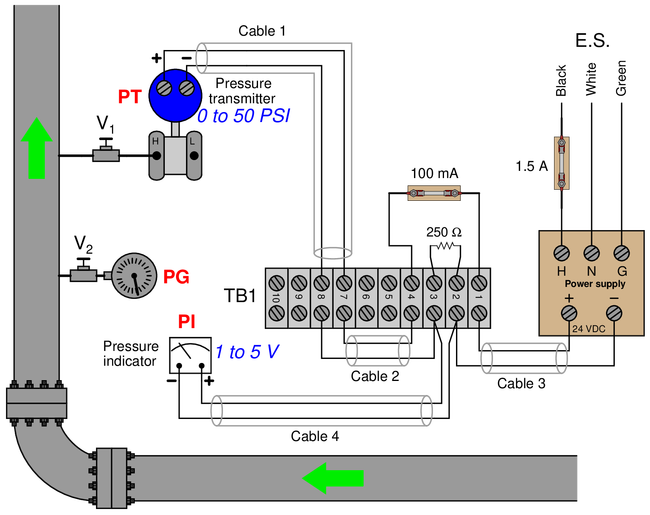Identify the likelihood of each specified fault for this circuit. Consider each fault one at a time (i.e. no coincidental faults), determining whether or not each fault could independently account for {\it all} measurements and symptoms in this circuit.

$$\begin{array} {|l|l|} \hline Fault & Possible & Impossible \\ \hline 250 ohm resistor failed open & & \\ \hline 100 mA fuse blown & & \\ \hline 1.5 A fuse blown & & \\ \hline Cable 1 failed open & & \\ \hline Cable 3 failed open & & \\ \hline Cable 1 failed shorted & & \\ \hline Cable 4 failed shorted & & \\ \hline \end{array}$$

• #### Question 35

This pressure-measurement system seems to have a problem. The pressure gauge (PG) indicates 30 PSI, but the pressure indicator (PI) reads over 50 PSI (pegged’’ fully up-scale). A voltmeter connected between terminals 4 and 2 registers 24 VDC:Identify the likelihood of each specified fault for this circuit. Consider each fault one at a time (i.e. no coincidental faults), determining whether or not each fault could independently account for {\it all} measurements and symptoms in this circuit.

$$\begin{array} {|l|l|} \hline Fault & Possible & Impossible \\ \hline 250 ohm resistor failed open & & \\ \hline 100 mA fuse blown & & \\ \hline 1.5 A fuse blown & & \\ \hline Cable 1 failed open & & \\ \hline Cable 4 failed open & & \\ \hline Cable 1 failed shorted & & \\ \hline Cable 4 failed shorted & & \\ \hline \end{array}$$

• #### Question 36

This pressure-measurement system seems to have a problem. The pressure gauge (PG) indicates 30 PSI, but the pressure indicator (PI) reads less than 0 PSI (pegged’’ fully down-scale). A voltmeter connected between terminals 7 and 8 registers 0 VDC:Identify the likelihood of each specified fault for this circuit. Consider each fault one at a time (i.e. no coincidental faults), determining whether or not each fault could independently account for {\it all} measurements and symptoms in this circuit.

$$\begin{array} {|l|l|} \hline Fault & Possible & Impossible \\ \hline 250 ohm resistor failed open & & \\ \hline 100 mA fuse blown & & \\ \hline 1.5 A fuse blown & & \\ \hline Cable 1 failed open & & \\ \hline Cable 4 failed open & & \\ \hline Cable 1 failed shorted & & \\ \hline Cable 4 failed shorted & & \\ \hline \end{array}$$

• #### Question 37

This pictorial diagram shows the wiring connections for a simple pressure control loop, where a loop-powered 4-20 mA pressure transmitter sends a signal to a Honeywell controller, which in turn sends another 4-20 mA signal to a control valve: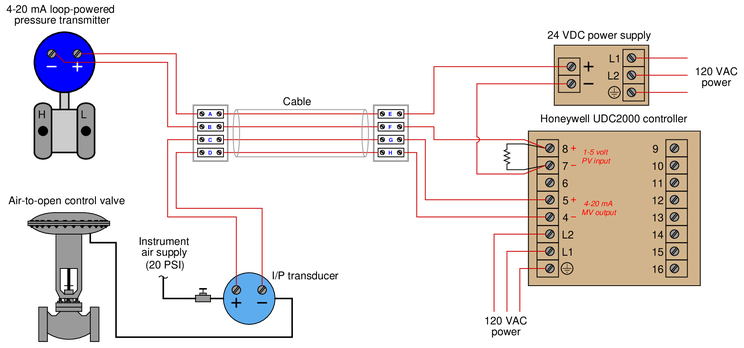If an operator informs you that the control valve refuses to open even in manual mode, what types and locations of electrical faults might you suspect? Are there any non-electrical faults which might also cause this to happen?

• #### Question 38

This pictorial diagram shows the wiring connections for a simple pressure control loop, where a loop-powered 4-20 mA pressure transmitter sends a signal to a Honeywell controller, which in turn sends another 4-20 mA signal to a control valve:If an operator informs you that the pressure indicated by the Honeywell controller is above range (pegged’’ full upscale, reading +110

• #### Question 39

Suppose a voltmeter registers 0 volts between test points {\bf C} and {\bf F} in this series-parallel circuit:Identify the likelihood of each specified fault for this circuit. Consider each fault one at a time (i.e. no coincidental faults), determining whether or not each fault could independently account for {\it all} measurements and symptoms in this circuit.

$$\begin{array} {|l|l|} \hline Fault & Possible & Impossible \\ \hline R_1 failed open & & \\ \hline R_2 failed open & & \\ \hline R_3 failed open & & \\ \hline R_1 failed shorted & & \\ \hline R_2 failed shorted & & \\ \hline R_3 failed shorted & & \\ \hline Voltage source dead & & \\ \hline \end{array}$$

• #### Question 40

Suppose an ammeter inserted between test points {\bf E} and {\bf F} registers 4 mA in this series-parallel circuit:Identify the likelihood of each specified fault for this circuit. Consider each fault one at a time (i.e. no coincidental faults), determining whether or not each fault could independently account for {\it all} measurements and symptoms in this circuit.

$$\begin{array} {|l|l|} \hline Fault & Possible & Impossible \\ \hline R_1 failed open & & \\ \hline R_2 failed open & & \\ \hline R_3 failed open & & \\ \hline R_1 failed shorted & & \\ \hline R_2 failed shorted & & \\ \hline R_3 failed shorted & & \\ \hline Current source dead & & \\ \hline \end{array}$$

• #### Question 41

Suppose an ammeter inserted between test points {\bf B} and {\bf D} registers 0 mA in this series-parallel circuit:Identify the likelihood of each specified fault for this circuit. Consider each fault one at a time (i.e. no coincidental faults), determining whether or not each fault could independently account for {\it all} measurements and symptoms in this circuit.

$$\begin{array} {|l|l|} \hline Fault & Possible & Impossible \\ \hline R_1 failed open & & \\ \hline R_2 failed open & & \\ \hline R_3 failed open & & \\ \hline R_1 failed shorted & & \\ \hline R_2 failed shorted & & \\ \hline R_3 failed shorted & & \\ \hline Current source dead & & \\ \hline \end{array}$$

• #### Question 42

Suppose a voltmeter registers 0 volts between test points {\bf C} and {\bf D} in this series-parallel circuit:Identify the likelihood of each specified fault for this circuit. Consider each fault one at a time (i.e. no coincidental faults), determining whether or not each fault could independently account for {\it all} measurements and symptoms in this circuit.

$$\begin{array} {|l|l|} \hline Fault & Possible & Impossible \\ \hline R_1 failed open & & \\ \hline R_2 failed open & & \\ \hline R_3 failed open & & \\ \hline R_1 failed shorted & & \\ \hline R_2 failed shorted & & \\ \hline R_3 failed shorted & & \\ \hline Current source dead & & \\ \hline \end{array}$$

• #### Question 43

Suppose an ammeter inserted between test points {\bf E} and {\bf C} registers 6 mA in this series-parallel circuit:Identify the likelihood of each specified fault for this circuit. Consider each fault one at a time (i.e. no coincidental faults), determining whether or not each fault could independently account for {\it all} measurements and symptoms in this circuit.

$$\begin{array} {|l|l|} \hline Fault & Possible & Impossible \\ \hline R_1 failed open & & \\ \hline R_2 failed open & & \\ \hline R_3 failed open & & \\ \hline R_1 failed shorted & & \\ \hline R_2 failed shorted & & \\ \hline R_3 failed shorted & & \\ \hline Current source dead & & \\ \hline \end{array}$$

• #### Question 44

Suppose a voltmeter registers 8 volts between test points {\bf A} and {\bf F} in this series-parallel circuit:Identify the likelihood of each specified fault for this circuit. Consider each fault one at a time (i.e. no coincidental faults), determining whether or not each fault could independently account for {\it all} measurements and symptoms in this circuit.

$$\begin{array} {|l|l|} \hline Fault & Possible & Impossible \\ \hline R_1 failed open & & \\ \hline R_2 failed open & & \\ \hline R_3 failed open & & \\ \hline R_1 failed shorted & & \\ \hline R_2 failed shorted & & \\ \hline R_3 failed shorted & & \\ \hline Current source dead & & \\ \hline \end{array}$$

• #### Question 45

Suppose an ammeter inserted between test point {\bf D} and the nearest lead of resistor R_2 registers 0 mA in this series-parallel circuit:Identify the likelihood of each specified fault for this circuit. Consider each fault one at a time (i.e. no coincidental faults), determining whether or not each fault could independently account for {\it all} measurements and symptoms in this circuit.

$$\begin{array} {|l|l|} \hline Fault & Possible & Impossible \\ \hline R_1 failed open & & \\ \hline R_2 failed open & & \\ \hline R_3 failed open & & \\ \hline R_1 failed shorted & & \\ \hline R_2 failed shorted & & \\ \hline R_3 failed shorted & & \\ \hline Current source dead & & \\ \hline \end{array}$$

• #### Question 46

Suppose an ammeter inserted between test point {\bf C} and the nearest lead of resistor R_3 registers 4 mA in this series-parallel circuit:Identify the likelihood of each specified fault for this circuit. Consider each fault one at a time (i.e. no coincidental faults), determining whether or not each fault could independently account for {\it all} measurements and symptoms in this circuit.

$$\begin{array} {|l|l|} \hline Fault & Possible & Impossible \\ \hline R_1 failed open & & \\ \hline R_2 failed open & & \\ \hline R_3 failed open & & \\ \hline R_1 failed shorted & & \\ \hline R_2 failed shorted & & \\ \hline R_3 failed shorted & & \\ \hline Current source dead & & \\ \hline \end{array}$$

• #### Question 47

Suppose an ammeter inserted between test point {\bf C} and the nearest lead of resistor R_1 registers 0 mA in this series-parallel circuit:Identify the likelihood of each specified fault for this circuit. Consider each fault one at a time (i.e. no coincidental faults), determining whether or not each fault could independently account for {\it all} measurements and symptoms in this circuit.

$$\begin{array} {|l|l|} \hline Fault & Possible & Impossible \\ \hline R_1 failed open & & \\ \hline R_2 failed open & & \\ \hline R_3 failed open & & \\ \hline R_1 failed shorted & & \\ \hline R_2 failed shorted & & \\ \hline R_3 failed shorted & & \\ \hline Voltage source dead & & \\ \hline \end{array}$$

• #### Question 48

Suppose a voltmeter registers 6 volts between test points {\bf E} and {\bf C} in this series-parallel circuit:Identify the likelihood of each specified fault for this circuit. Consider each fault one at a time (i.e. no coincidental faults), determining whether or not each fault could independently account for {\it all} measurements and symptoms in this circuit.

$$\begin{array} {|l|l|} \hline Fault & Possible & Impossible \\ \hline R_1 failed open & & \\ \hline R_2 failed open & & \\ \hline R_3 failed open & & \\ \hline R_1 failed shorted & & \\ \hline R_2 failed shorted & & \\ \hline R_3 failed shorted & & \\ \hline Voltage source dead & & \\ \hline \end{array}$$

• #### Question 49

Suppose an ammeter inserted between test points {\bf D} and {\bf B} registers 16 mA in this series-parallel circuit:Identify the likelihood of each specified fault for this circuit. Consider each fault one at a time (i.e. no coincidental faults), determining whether or not each fault could independently account for {\it all} measurements and symptoms in this circuit.

$$\begin{array} {|l|l|} \hline Fault & Possible & Impossible \\ \hline R_1 failed open & & \\ \hline R_2 failed open & & \\ \hline R_3 failed open & & \\ \hline R_1 failed shorted & & \\ \hline R_2 failed shorted & & \\ \hline R_3 failed shorted & & \\ \hline Voltage source dead & & \\ \hline \end{array}$$

• #### Question 50

Suppose a voltmeter registers 12 volts between test points {\bf C} and {\bf D} in this series-parallel circuit:Identify the likelihood of each specified fault for this circuit. Consider each fault one at a time (i.e. no coincidental faults), determining whether or not each fault could independently account for {\it all} measurements and symptoms in this circuit.

$$\begin{array} {|l|l|} \hline Fault & Possible & Impossible \\ \hline R_1 failed open & & \\ \hline R_2 failed open & & \\ \hline R_3 failed open & & \\ \hline R_1 failed shorted & & \\ \hline R_2 failed shorted & & \\ \hline R_3 failed shorted & & \\ \hline Voltage source dead & & \\ \hline \end{array}$$

• #### Question 51

Suppose a voltmeter registers 6 volts between test points {\bf C} and {\bf B} in this series-parallel circuit:Identify the likelihood of each specified fault for this circuit. Consider each fault one at a time (i.e. no coincidental faults), determining whether or not each fault could independently account for {\it all} measurements and symptoms in this circuit.

$$\begin{array} {|l|l|} \hline Fault & Possible & Impossible \\ \hline R_1 failed open & & \\ \hline R_2 failed open & & \\ \hline R_3 failed open & & \\ \hline R_1 failed shorted & & \\ \hline R_2 failed shorted & & \\ \hline R_3 failed shorted & & \\ \hline Voltage source dead & & \\ \hline \end{array}$$

• #### Question 52

Suppose a voltmeter registers 18 volts between test points {\bf E} and {\bf C} while the pushbutton is released (not pressed), and 0 volts between test points {\bf A} and {\bf B} while the pushbutton is pressed:Identify the likelihood of each specified fault for this circuit. Consider each fault one at a time (i.e. no coincidental faults), determining whether or not each fault could independently account for {\it all} measurements and symptoms in this circuit.

$$\begin{array} {|l|l|} \hline Fault & Possible & Impossible \\ \hline Switch failed open & & \\ \hline R_1 failed open & & \\ \hline R_2 failed open & & \\ \hline R_3 failed open & & \\ \hline Switch failed shorted & & \\ \hline R_1 failed shorted & & \\ \hline R_2 failed shorted & & \\ \hline R_3 failed shorted & & \\ \hline Voltage source dead & & \\ \hline \end{array}$$

• #### Question 53

Suppose a voltmeter registers 0 volts between test points {\bf E} and {\bf C} while the pushbutton is released (not pressed), and 0 volts between test points {\bf B} and {\bf D} while the pushbutton is pressed:Identify the likelihood of each specified fault for this circuit. Consider each fault one at a time (i.e. no coincidental faults), determining whether or not each fault could independently account for {\it all} measurements and symptoms in this circuit.

$$\begin{array} {|l|l|} \hline Fault & Possible & Impossible \\ \hline Switch failed open & & \\ \hline R_1 failed open & & \\ \hline R_2 failed open & & \\ \hline R_3 failed open & & \\ \hline Switch failed shorted & & \\ \hline R_1 failed shorted & & \\ \hline R_2 failed shorted & & \\ \hline R_3 failed shorted & & \\ \hline Voltage source dead & & \\ \hline \end{array}$$

• #### Question 54

Suppose the lamp refuses to light up when the pushbutton switch is pressed. A voltmeter registers 12 volts between test points {\bf C} and {\bf E} in the circuit while the pushbutton is released (not pressed):Identify the likelihood of each specified fault for this circuit. Consider each fault one at a time (i.e. no coincidental faults), determining whether or not each fault could independently account for {\it all} measurements and symptoms in this circuit.

$$\begin{array} {|l|l|} \hline Fault & Possible & Impossible \\ \hline Open wire between A and C & & \\ \hline Open wire between B and D & & \\ \hline Open wire between D and F & & \\ \hline Lamp failed open & & \\ \hline Switch failed open & & \\ \hline Lamp failed shorted & & \\ \hline Switch failed shorted & & \\ \hline Voltage source dead & & \\ \hline \end{array}$$

• #### Question 55

Suppose the lamp refuses to light up when the pushbutton switch is pressed. A voltmeter registers 0 volts between test points {\bf E} and {\bf D} in the circuit while the pushbutton is pressed:Identify the likelihood of each specified fault for this circuit. Consider each fault one at a time (i.e. no coincidental faults), determining whether or not each fault could independently account for {\it all} measurements and symptoms in this circuit.

$$\begin{array} {|l|l|} \hline Fault & Possible & Impossible \\ \hline Open wire between A and C & & \\ \hline Open wire between B and D & & \\ \hline Open wire between D and F & & \\ \hline Lamp failed open & & \\ \hline Switch failed open & & \\ \hline Lamp failed shorted & & \\ \hline Switch failed shorted & & \\ \hline Voltage source dead & & \\ \hline \end{array}$$

• #### Question 56

Suppose the lamp refuses to light up when the pushbutton switch is pressed. A voltmeter registers 0 volts between test points {\bf A} and {\bf D} in the circuit while the pushbutton is pressed:Identify the likelihood of each specified fault for this circuit. Consider each fault one at a time (i.e. no coincidental faults), determining whether or not each fault could independently account for {\it all} measurements and symptoms in this circuit.

$$\begin{array} {|l|l|} \hline Fault & Possible & Impossible \\ \hline Open wire between A and C & & \\ \hline Open wire between B and D & & \\ \hline Open wire between D and F & & \\ \hline Lamp failed open & & \\ \hline Switch failed open & & \\ \hline Lamp failed shorted & & \\ \hline Switch failed shorted & & \\ \hline Voltage source dead & & \\ \hline \end{array}$$

• #### Question 57

Identify which of these are true statements:

{1.} Between two points that are electrically common to each other, there is guaranteed to be zero (or nearly zero) voltage.

{2.} If zero voltage is measured between two points, those points must be electrically common to each other.

{3.} Between two points that are not electrically common to each other, there is guaranteed to be voltage.

{4.} If voltage is measured between two points, those points cannot be electrically common to each other.

• #### Question 58

Suppose a voltmeter registers 4 volts between test points {\bf E} and {\bf C} in this series-parallel circuit:Identify the likelihood of each specified fault for this circuit. Consider each fault one at a time (i.e. no coincidental faults), determining whether or not each fault could independently account for {\it all} measurements and symptoms in this circuit.

$$\begin{array} {|l|l|} \hline Fault & Possible & Impossible \\ \hline R_1 failed open & & \\ \hline R_2 failed open & & \\ \hline R_3 failed open & & \\ \hline R_1 failed shorted & & \\ \hline R_2 failed shorted & & \\ \hline R_3 failed shorted & & \\ \hline Current source dead & & \\ \hline \end{array}$$

• #### Question 59

Suppose a voltmeter registers 12 volts between test points {\bf D} and {\bf A} in this series-parallel circuit:Identify the likelihood of each specified fault for this circuit. Consider each fault one at a time (i.e. no coincidental faults), determining whether or not each fault could independently account for {\it all} measurements and symptoms in this circuit.

$$\begin{array} {|l|l|} \hline Fault & Possible & Impossible \\ \hline R_1 failed open & & \\ \hline R_2 failed open & & \\ \hline R_3 failed open & & \\ \hline R_4 failed open & & \\ \hline R_1 failed shorted & & \\ \hline R_2 failed shorted & & \\ \hline R_3 failed shorted & & \\ \hline R_4 failed shorted & & \\ \hline Current source dead & & \\ \hline \end{array}$$

• #### Question 60

Suppose a voltmeter registers 13.33 volts between test points {\bf D} and {\bf E} in this series-parallel circuit:Identify the likelihood of each specified fault for this circuit. Consider each fault one at a time (i.e. no coincidental faults), determining whether or not each fault could independently account for {\it all} measurements and symptoms in this circuit.

$$\begin{array} {|l|l|} \hline Fault & Possible & Impossible \\ \hline R_1 failed open & & \\ \hline R_2 failed open & & \\ \hline R_3 failed open & & \\ \hline R_4 failed open & & \\ \hline R_1 failed shorted & & \\ \hline R_2 failed shorted & & \\ \hline R_3 failed shorted & & \\ \hline R_4 failed shorted & & \\ \hline Voltage source dead & & \\ \hline \end{array}$$

• #### Question 61

Suppose a voltmeter registers 12 volts between test points {\bf A} and {\bf D} in this series-parallel circuit:Identify the likelihood of each specified fault for this circuit. Consider each fault one at a time (i.e. no coincidental faults), determining whether or not each fault could independently account for {\it all} measurements and symptoms in this circuit.

$$\begin{array} {|l|l|} \hline Fault & Possible & Impossible \\ \hline R_1 failed open & & \\ \hline R_2 failed open & & \\ \hline R_3 failed open & & \\ \hline R_4 failed open & & \\ \hline R_1 failed shorted & & \\ \hline R_2 failed shorted & & \\ \hline R_3 failed shorted & & \\ \hline R_4 failed shorted & & \\ \hline Voltage source dead & & \\ \hline \end{array}$$

• #### Question 62

Suppose a voltmeter registers 6 volts between test points {\bf D} and {\bf E} in this series-parallel circuit:Identify the likelihood of each specified fault for this circuit. Consider each fault one at a time (i.e. no coincidental faults), determining whether or not each fault could independently account for {\it all} measurements and symptoms in this circuit.

$$\begin{array} {|l|l|} \hline Fault & Possible & Impossible \\ \hline R_1 failed open & & \\ \hline R_2 failed open & & \\ \hline R_3 failed open & & \\ \hline R_4 failed open & & \\ \hline R_1 failed shorted & & \\ \hline R_2 failed shorted & & \\ \hline R_3 failed shorted & & \\ \hline R_4 failed shorted & & \\ \hline Voltage source dead & & \\ \hline \end{array}$$

• #### Question 63

Suppose a voltmeter registers 0 volts between test points {\bf F} and {\bf E} in this series-parallel circuit:Identify the likelihood of each specified fault for this circuit. Consider each fault one at a time (i.e. no coincidental faults), determining whether or not each fault could independently account for {\it all} measurements and symptoms in this circuit.

$$\begin{array} {|l|l|} \hline Fault & Possible & Impossible \\ \hline R_1 failed open & & \\ \hline R_2 failed open & & \\ \hline R_3 failed open & & \\ \hline R_4 failed open & & \\ \hline R_1 failed shorted & & \\ \hline R_2 failed shorted & & \\ \hline R_3 failed shorted & & \\ \hline R_4 failed shorted & & \\ \hline Voltage source dead & & \\ \hline \end{array}$$

• #### Question 64

Suppose a voltmeter registers 20 volts between test points {\bf D} and {\bf E} in this series-parallel circuit:Identify the likelihood of each specified fault for this circuit. Consider each fault one at a time (i.e. no coincidental faults), determining whether or not each fault could independently account for {\it all} measurements and symptoms in this circuit.

$$\begin{array} {|l|l|} \hline Fault & Possible & Impossible \\ \hline R_1 failed open & & \\ \hline R_2 failed open & & \\ \hline R_3 failed open & & \\ \hline R_4 failed open & & \\ \hline R_1 failed shorted & & \\ \hline R_2 failed shorted & & \\ \hline R_3 failed shorted & & \\ \hline R_4 failed shorted & & \\ \hline Voltage source dead & & \\ \hline \end{array}$$

• #### Question 65

Suppose a voltmeter registers 0 volts between test points {\bf A} and {\bf D} in this series-parallel circuit:Identify the likelihood of each specified fault for this circuit. Consider each fault one at a time (i.e. no coincidental faults), determining whether or not each fault could independently account for {\it all} measurements and symptoms in this circuit.

$$\begin{array} {|l|l|} \hline Fault & Possible & Impossible \\ \hline R_1 failed open & & \\ \hline R_2 failed open & & \\ \hline R_3 failed open & & \\ \hline R_4 failed open & & \\ \hline R_1 failed shorted & & \\ \hline R_2 failed shorted & & \\ \hline R_3 failed shorted & & \\ \hline R_4 failed shorted & & \\ \hline Voltage source dead & & \\ \hline \end{array}$$

• #### Question 66

Suppose an ammeter inserted between test point {\bf F} and the nearest lead of resistor R_3 registers 0 mA in this series-parallel circuit:Identify the likelihood of each specified fault for this circuit. Consider each fault one at a time (i.e. no coincidental faults), determining whether or not each fault could independently account for {\it all} measurements and symptoms in this circuit.

$$\begin{array} {|l|l|} \hline Fault & Possible & Impossible \\ \hline R_1 failed open & & \\ \hline R_2 failed open & & \\ \hline R_3 failed open & & \\ \hline R_1 failed shorted & & \\ \hline R_2 failed shorted & & \\ \hline R_3 failed shorted & & \\ \hline Current source dead & & \\ \hline \end{array}$$

• #### Question 67

Everything I know about troubleshooting, I learned from Tyler Durden (with respects to the movie {\it Fight Club}):

{The Rules of \underline{ Fault Club}}

(1) Don’t try to find the fault by looking for it—perform diagnostic tests instead

(2) {\it Don't try to find the fault by looking for it -- perform diagnostic tests instead!}

(3) The troubleshooting is over when you have correctly identified the nature and location of the fault

(4) It’s just you and the fault—don’t ask for help until you have exhausted your resources

(5) Assume one fault at a time in a proven system, unless the data proves otherwise

(6) No new components allowed—replacing suspected bad components with new is a waste of time and money

(7) We will practice as many times as we have to until you master this

(8) Troubleshooting is not a spectator sport:

For each of these rules, explain their rationale.

• #### Question 68

Suppose a voltmeter registers 0 volts between test points {\bf C} and {\bf E} in this series-parallel circuit:Identify the likelihood of each specified fault for this circuit. Consider each fault one at a time (i.e. no coincidental faults), determining whether or not each fault could independently account for {\it all} measurements and symptoms in this circuit.

$$\begin{array} {|l|l|} \hline Fault & Possible & Impossible \\ \hline R_1 failed open & & \\ \hline R_2 failed open & & \\ \hline R_3 failed open & & \\ \hline R_1 failed shorted & & \\ \hline R_2 failed shorted & & \\ \hline R_3 failed shorted & & \\ \hline Current source dead & & \\ \hline \end{array}$$

• #### Question 69

Suppose a voltmeter registers 4 volts between test points {\bf A} and {\bf C} in this series-parallel circuit:Identify the likelihood of each specified fault for this circuit. Consider each fault one at a time (i.e. no coincidental faults), determining whether or not each fault could independently account for {\it all} measurements and symptoms in this circuit.

$$\begin{array} {|l|l|} \hline Fault & Possible & Impossible \\ \hline R_1 failed open & & \\ \hline R_2 failed open & & \\ \hline R_3 failed open & & \\ \hline R_1 failed shorted & & \\ \hline R_2 failed shorted & & \\ \hline R_3 failed shorted & & \\ \hline Current source dead & & \\ \hline \end{array}$$

• #### Question 70

Suppose a voltmeter registers 24 volts between test points {\bf C} and {\bf A} in this series-parallel circuit:Identify the likelihood of each specified fault for this circuit. Consider each fault one at a time (i.e. no coincidental faults), determining whether or not each fault could independently account for {\it all} measurements and symptoms in this circuit.

$$\begin{array} {|l|l|} \hline Fault & Possible & Impossible \\ \hline R_1 failed open & & \\ \hline R_2 failed open & & \\ \hline R_3 failed open & & \\ \hline R_1 failed shorted & & \\ \hline R_2 failed shorted & & \\ \hline R_3 failed shorted & & \\ \hline Current source dead & & \\ \hline \end{array}$$

• #### Question 71

Suppose a voltmeter registers 0 volts between test points {\bf D} and {\bf B} in this series-parallel circuit:Identify the likelihood of each specified fault for this circuit. Consider each fault one at a time (i.e. no coincidental faults), determining whether or not each fault could independently account for {\it all} measurements and symptoms in this circuit.

$$\begin{array} {|l|l|} \hline Fault & Possible & Impossible \\ \hline R_1 failed open & & \\ \hline R_2 failed open & & \\ \hline R_3 failed open & & \\ \hline R_1 failed shorted & & \\ \hline R_2 failed shorted & & \\ \hline R_3 failed shorted & & \\ \hline Voltage source dead & & \\ \hline \end{array}$$

• #### Question 72

Suppose a voltmeter registers 18 volts between test points {\bf C} and {\bf E} in this series-parallel circuit:Identify the likelihood of each specified fault for this circuit. Consider each fault one at a time (i.e. no coincidental faults), determining whether or not each fault could independently account for {\it all} measurements and symptoms in this circuit.

$$\begin{array} {|l|l|} \hline Fault & Possible & Impossible \\ \hline R_1 failed open & & \\ \hline R_2 failed open & & \\ \hline R_3 failed open & & \\ \hline R_1 failed shorted & & \\ \hline R_2 failed shorted & & \\ \hline R_3 failed shorted & & \\ \hline Voltage source dead & & \\ \hline \end{array}$$

• #### Question 73

Suppose an ammeter inserted between test point {\bf D} and the nearest lead of voltage source registers 9 mA in this series-parallel circuit:Identify the likelihood of each specified fault for this circuit. Consider each fault one at a time (i.e. no coincidental faults), determining whether or not each fault could independently account for {\it all} measurements and symptoms in this circuit.

$$\begin{array} {|l|l|} \hline Fault & Possible & Impossible \\ \hline R_1 failed open & & \\ \hline R_2 failed open & & \\ \hline R_3 failed open & & \\ \hline R_1 failed shorted & & \\ \hline R_2 failed shorted & & \\ \hline R_3 failed shorted & & \\ \hline Voltage source dead & & \\ \hline \end{array}$$

• #### Question 74

Suppose an ammeter inserted between test point {\bf C} and the nearest lead of resistor R_2 registers 9 mA in this series-parallel circuit:Identify the likelihood of each specified fault for this circuit. Consider each fault one at a time (i.e. no coincidental faults), determining whether or not each fault could independently account for {\it all} measurements and symptoms in this circuit.

$$\begin{array} {|l|l|} \hline Fault & Possible & Impossible \\ \hline R_1 failed open & & \\ \hline R_2 failed open & & \\ \hline R_3 failed open & & \\ \hline R_1 failed shorted & & \\ \hline R_2 failed shorted & & \\ \hline R_3 failed shorted & & \\ \hline Voltage source dead & & \\ \hline \end{array}$$

• #### Question 75

Suppose a voltmeter registers 16 volts between test points {\bf F} and {\bf D} in this series-parallel circuit:Identify the likelihood of each specified fault for this circuit. Consider each fault one at a time (i.e. no coincidental faults), determining whether or not each fault could independently account for {\it all} measurements and symptoms in this circuit.

$$\begin{array} {|l|l|} \hline Fault & Possible & Impossible \\ \hline R_1 failed open & & \\ \hline R_2 failed open & & \\ \hline R_3 failed open & & \\ \hline R_1 failed shorted & & \\ \hline R_2 failed shorted & & \\ \hline R_3 failed shorted & & \\ \hline Voltage source dead & & \\ \hline \end{array}$$